# PSAT Math : General Fractions

## Example Questions

← Previous 1 3 4

### Example Question #1 : How To Find The Reciprocal Of A Fraction

What is the reciprocal of the following fraction: 18/27

-18/27

9

9/27

27/18

27/18

Explanation:

A fraction multiplied by its reciprocal will equal 1. To find the reciprocal of a fraction, switch the denominator and numerator. The reciprocal of 18/27 is 27/18.

### Example Question #1 : How To Find The Lowest / Least Common Denominator

3/5 + 4/7 – 1/3 =

3/37

4/3

7/9

72/89

88/105

88/105

Explanation:

We need to find a common denominator to add and subtract these fractions. Let's do the addition first. The lowest common denominator of 5 and 7 is 5 * 7 = 35, so 3/5 + 4/7 = 21/35 + 20/35 = 41/35.

Now to the subtraction. The lowest common denominator of 35 and 3 is 35 * 3 = 105, so altogether, 3/5 + 4/7 – 1/3 = 41/35 – 1/3 = 123/105 – 35/105 = 88/105. This does not simplify and is therefore the correct answer.

### Example Question #1 : General Fractions

3/5 + 4/7 – 1/3 =

3/37

4/3

88/105

72/89

7/9

88/105

Explanation:

We need to find a common denominator to add and subtract these fractions. Let's do the addition first. The lowest common denominator of 5 and 7 is 5 * 7 = 35, so 3/5 + 4/7 = 21/35 + 20/35 = 41/35.

Now to the subtraction. The lowest common denominator of 35 and 3 is 35 * 3 = 105, so altogether, 3/5 + 4/7 – 1/3 = 41/35 – 1/3 = 123/105 – 35/105 = 88/105. This does not simplify and is therefore the correct answer.

### Example Question #2 : How To Find The Lowest / Least Common Denominator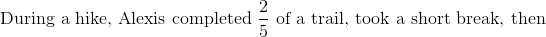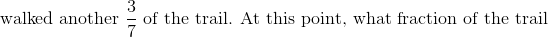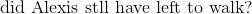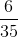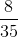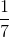Explanation: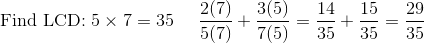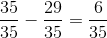### Example Question #3 : How To Find The Lowest / Least Common Denominator

Simplify: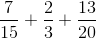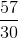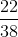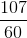Explanation:

First, find the common denominator of the fractions in order to add them together. Looking at the first two fractions, we can see that the common denominator is 15 because 3 times five is 15. We can now change both fractions to have a denominator of 15 so that we can add them together: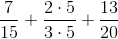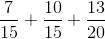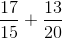Now, find a common denominator for the remaining fractions. This can be done by listing multiples of 15 and 20 and finding the lowest common multiple:

15: 15, 30, 45, 60, 75

20: 20, 40, 60, 80

60 is the lowest common multiple, so it is our least common denominator.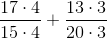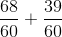### Example Question #1 : General Fractions

Simplify: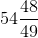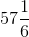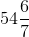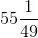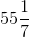Explanation: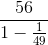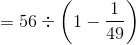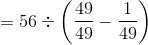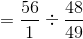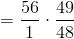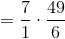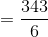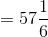### Example Question #2 : General Fractions

Simplify: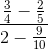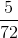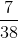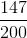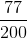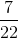Explanation: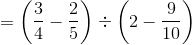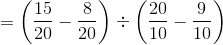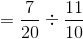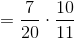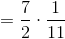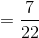### Example Question #3 : General Fractions

Simplify: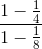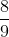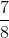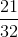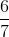Explanation: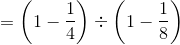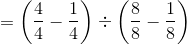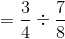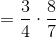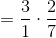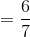### Example Question #4 : General Fractions

Simplify: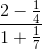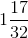None of the other responses gives the correct answer.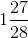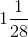Explanation: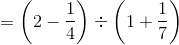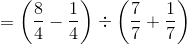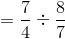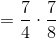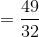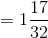### Example Question #5 : General Fractions

Simplify: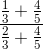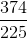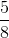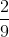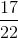Explanation: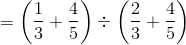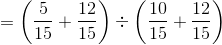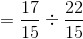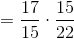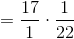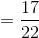← Previous 1 3 4